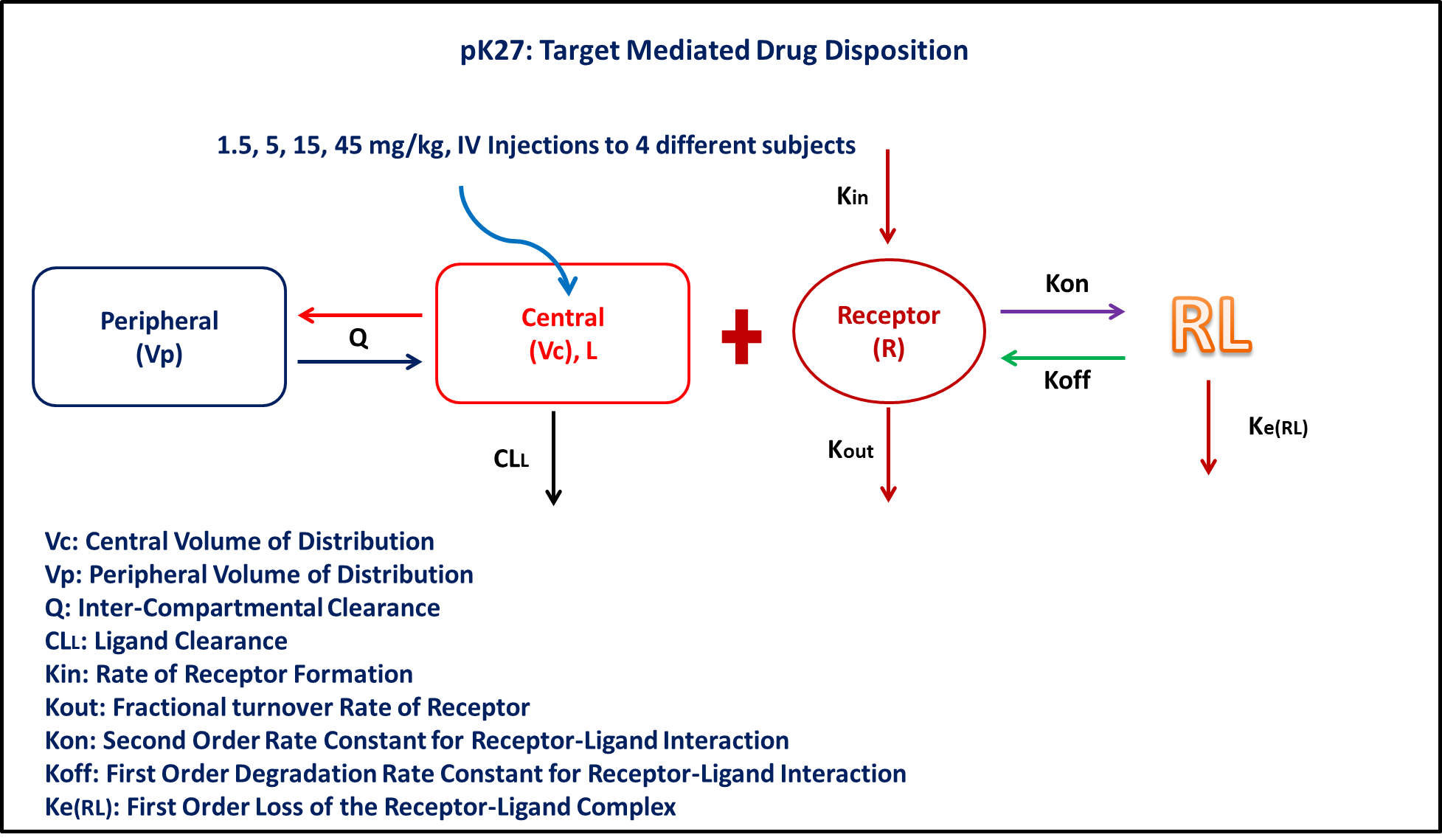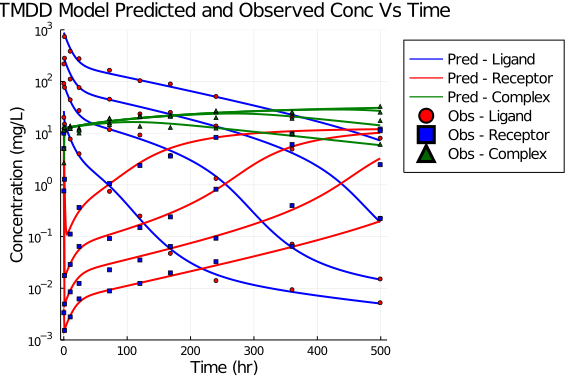# Exercise PK-27 - Target Mediated Drug Disposition

### Background

• Structural model - Target Mediated Drug Disposition Model (TMDD)

• Route of administration - IV-Bolus

• Dosage Regimen - 1.5, 5, 15, 45 mg/kg adminstered after complete washout

• Number of Subjects - 4### Learning Outcome

• To fit a full TMDD model with data from only ligand, ligand and target, target and ligand-target complex

• Write a differential equation for a full TMDD model

### Objective

• The objective of this exericse is to fit the data to a TMDD model

### Libraries

call the "necessary" libraries to get started

using Pumas
using Plots
using CSV
using StatsPlots
using Random


### Model

The given data follows a Target Mediated Drug Disposition Model

pk_27           = @model begin
@param begin
tvcl        ∈ RealDomain(lower=0)
tvkon       ∈ RealDomain(lower=0)
tvkoff      ∈ RealDomain(lower=0)
tvvp        ∈ RealDomain(lower=0)
tvq         ∈ RealDomain(lower=0)
tvkin       ∈ RealDomain(lower=0)
tvkout      ∈ RealDomain(lower=0)
tvkerl      ∈ RealDomain(lower=0)
tvvc        ∈ RealDomain(lower=0)
Ω           ∈ PDiagDomain(9)
σ²_prop_cp  ∈ RealDomain(lower=0)
σ²_prop_rec ∈ RealDomain(lower=0)
σ²_prop_com ∈ RealDomain(lower=0)
end

@random begin
η           ~ MvNormal(Ω)
end

@pre begin
Cl          = tvcl * exp(η)
Kon         = tvkon * exp(η)
Koff        = tvkoff * exp(η)
Vp          = tvvp * exp(η)
Q           = tvq * exp(η)
Kin         = tvkin * exp(η)
Kout        = tvkout * exp(η)
Kerl        = tvkerl * exp(η)
Vc          = tvvc * exp(η)
bioav       = (Central = 1/Vc,)
end

@init begin
Receptor    = Kin/Kout
end

@dynamics begin
Central'    = -(Cl/Vc)*Central - (Q/Vc)*Central + (Q/Vp)*Peripheral - Kon*Receptor*Central + Koff*Complex
Peripheral' = (Q/Vc)*Central - (Q/Vp)*Peripheral
Receptor'   = Kin - Kout*Receptor - Kon*Receptor*Central + Koff*Complex
Complex'    = Kon*Receptor*Central - Koff*Complex - Kerl*Complex
end

@derived begin
cp          = @. Central
dv_cp       = @. Normal(cp, sqrt(cp^2*σ²_prop_cp))
rec         = @. Receptor
dv_rec      = @. Normal(rec, sqrt(rec^2*σ²_prop_rec))
com         = @. Complex
dv_com      = @. Normal(com, sqrt(com^2*σ²_prop_com))
end
end

PumasModel
Parameters: tvcl, tvkon, tvkoff, tvvp, tvq, tvkin, tvkout, tvkerl, tvvc,
Ω, σ²_prop_cp, σ²_prop_rec, σ²_prop_com
Random effects: η
Covariates:
Dynamical variables: Central, Peripheral, Receptor, Complex
Derived: cp, dv_cp, rec, dv_rec, com, dv_com
Observed: cp, dv_cp, rec, dv_rec, com, dv_com


### Parameters

The parameters are as given below. tv represents the typical value for parameters.

• Cl - Clearance of central compartment (L/kg/hr)

• Kon - Second oder on rate of ligand (L/mg/hr)

• Koff - First order off rate of ligand (1/hr)

• Vp - Volume of Peripheral Compartment (L/kg)

• Q - Inter-compartmental clearance (L/kg/hr)

• Kin - Zero order receptor synthesis process (mg/L/hr)

• Kout - First order receptor degenration process (1/hr)

• Kerl - First order elimination of complex (1/hr)

• Vc - Volume of Central Compartment (L/kg)

param = ( tvcl        = 0.001,
tvkon       = 0.096,
tvkoff      = 0.001,
tvvp        = 0.100,
tvq         = 0.003,
tvkin       = 0.11,
tvkout      = 0.0089,
tvkerl      = 0.003,
tvvc        = 0.05,
Ω           = Diagonal([0.0,0.0,0.0,0.0,0.0,0.0,0.0,0.0,0.0]),
σ²_prop_cp  = 0.02,
σ²_prop_rec = 0.012,
σ²_prop_com = 0.015)

(tvcl = 0.001, tvkon = 0.096, tvkoff = 0.001, tvvp = 0.1, tvq = 0.003, tvki
n = 0.11, tvkout = 0.0089, tvkerl = 0.003, tvvc = 0.05, Ω = [0.0 0.0 … 0.0
0.0; 0.0 0.0 … 0.0 0.0; … ; 0.0 0.0 … 0.0 0.0; 0.0 0.0 … 0.0 0.0], σ²_prop_
cp = 0.02, σ²_prop_rec = 0.012, σ²_prop_com = 0.015)


### Dosage Regimen

• Single dose of 1.5 mg/kg was administered as IV-Bolus at time=0

• Single dose of 5 mg/kg was administered as IV-Bolus at time=0

• Single dose of 15 mg/kg was administered as IV-Bolus at time=0

• Single dose of 45 mg/kg was administered as IV-Bolus at time=0

ev1      = DosageRegimen(1.5, cmt=1, time=0)
sub1     = Subject(id=1, events=ev1)
ev2      = DosageRegimen(5, cmt=1, time=0)
sub2     = Subject(id=2, events=ev2)
ev3      = DosageRegimen(15, cmt=1, time=0)
sub3     = Subject(id=3, events=ev3)
ev4      = DosageRegimen(45, cmt=1, time=0)
sub4     = Subject(id=4, events=ev4)
pop4_sub = [sub1,sub2,sub3,sub4]

Population
Subjects: 4
Covariates:


### Simulation

We will simulate the full model and specific time points to be scattered on the data

Random.seed!(123)
sim_pop4 = simobs(pk_27, pop4_sub, param, obstimes=0.1:1:500)
df1      = DataFrame(sim_pop4)


### Dataframe and Plot

Convert the simulation to a dataframe and make necessary plots using dataframe

df1_dv = filter( x -> x.time in [0.1,1.1,10.1,24.1,72.1,120.1,168.1,240.1,360.1,499.1], df1)

@df df1 plot(:time, :cp, yaxis=:log, color=[:blue],
yticks=[0.001,0.01,0.1,1,10,100,1000], ylims=(0.001,1000), xlims=(-5,510),
legend=:outertopright, legendfontsize=10, titlefontsize=13,
xlabel="Time (hr)", ylabel="Concentration (mg/L)", linewidth=2,
title="TMDD Model Predicted and Observed Conc Vs Time", label="Pred - Ligand")
@df df1 plot!(:time, :rec, colour=[:red], label="Pred - Receptor", linewidth=2)
@df df1 plot!(:time, :com, color=[:green], label="Pred - Complex", linewidth=2)
@df df1_dv scatter!(:time, :dv_cp, color=[:red], label="Obs - Ligand", markershape=[:circle], markersize=3)
@df df1_dv scatter!(:time, :dv_rec, color=[:blue], label="Obs - Receptor", markershape=[:square], markersize=2)
@df df1_dv scatter!(:time, :dv_com, color=[:darkgreen], label="Obs - Complex", markershape=[:utriangle], markersize=2)